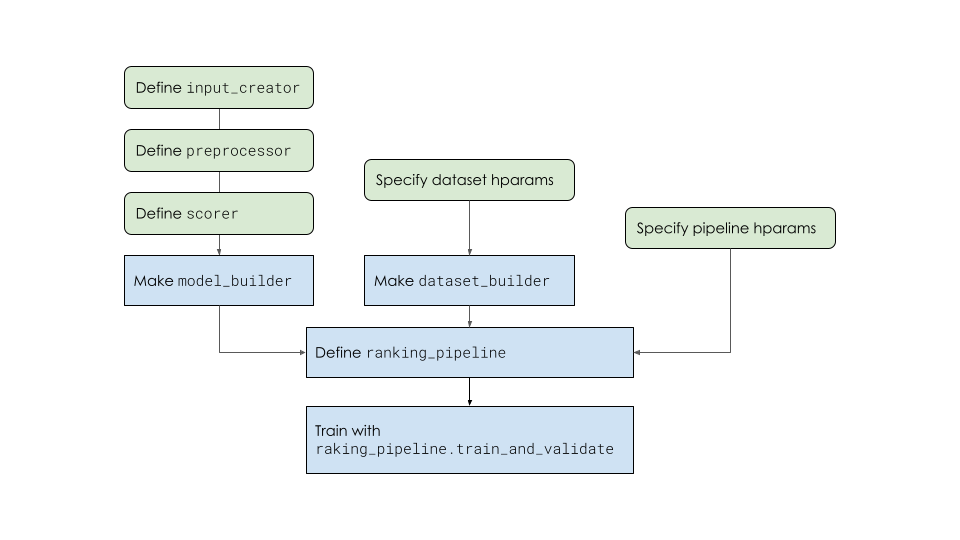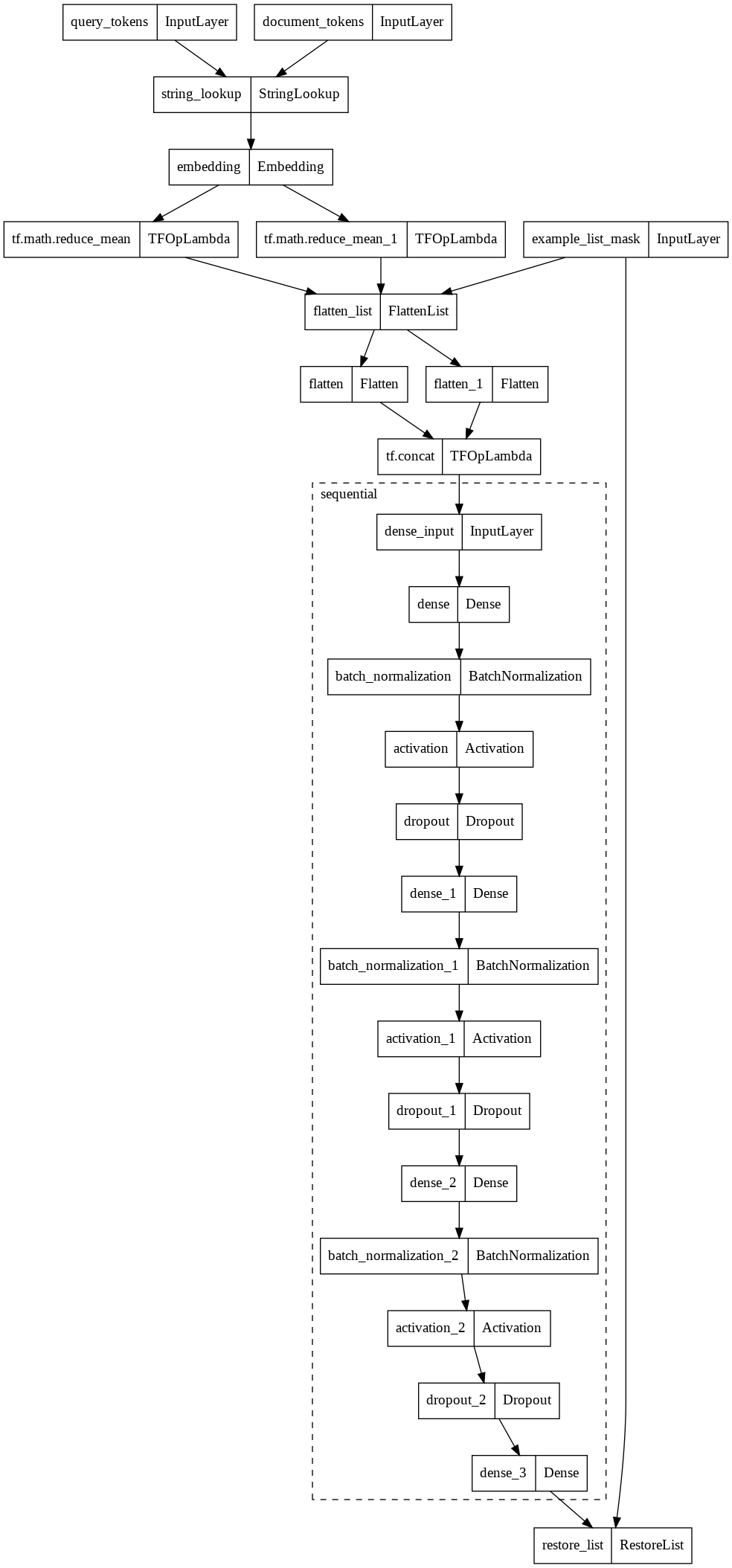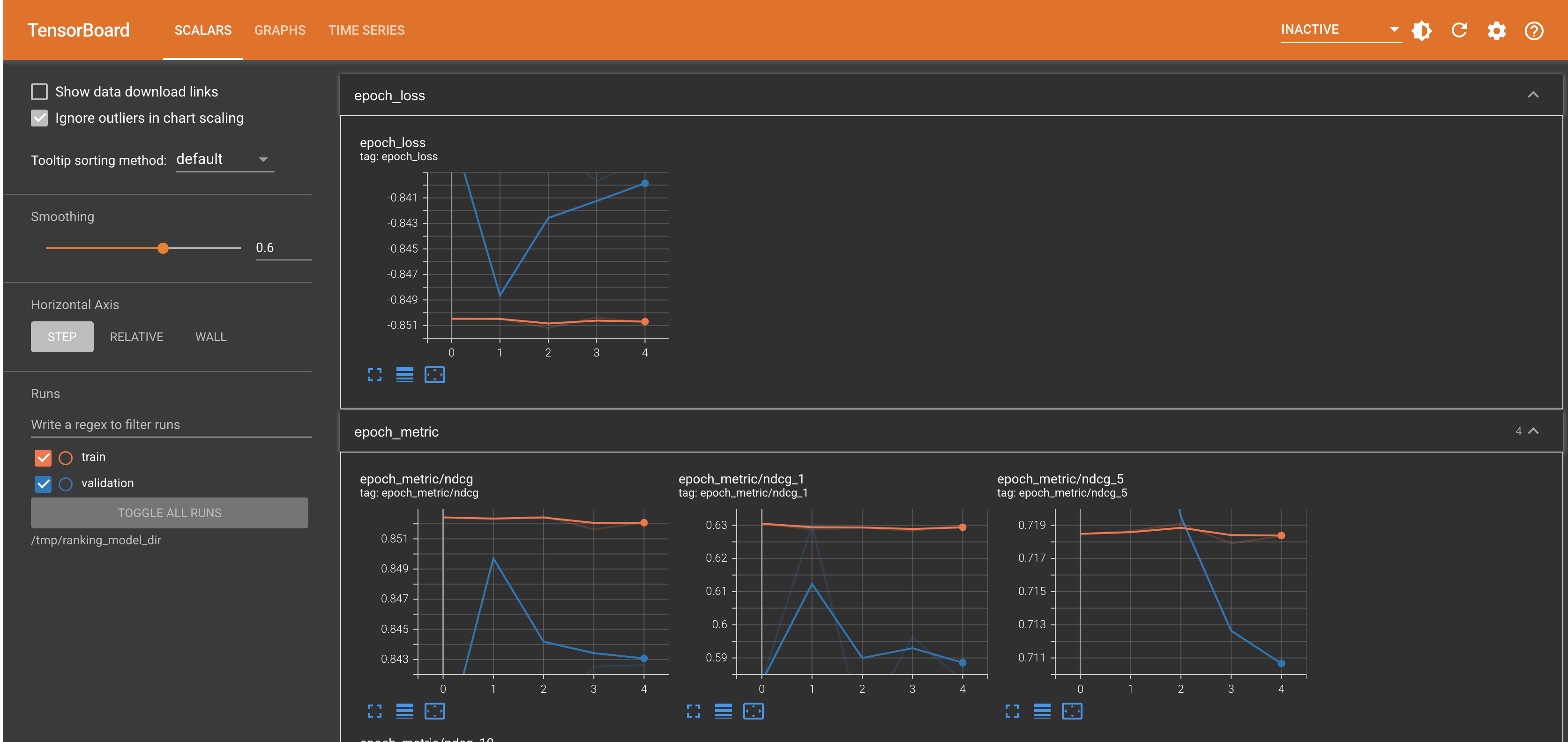Help protect the Great Barrier Reef with TensorFlow on Kaggle

TensorFlow Ranking Keras pipeline for distributed training

TensorFlow Ranking can handle heterogeneous dense and sparse features, and scales up to millions of data points. However, building and deploying a learning to rank model to operate at scale creates additional challenges beyond simply designing a model. The Ranking library provides workflow utility classes for building distributed training for large-scale ranking applications. For more information about these features, see the TensorFlow Ranking Overview.

This tutorial shows you how to build a ranking model that enables a distributed processing strategy by using the Ranking library's support for a pipeline processing architecture.

ANTIQUE dataset

In this tutorial, you will build a ranking model for ANTIQUE, a question-answering dataset. Given a query, and a list of answers, the objective is to rank the answers with optimal rank related metrics, such as NDCG. For more details about ranking metrics, review evaluation measures offline metrics.

ANTIQUE is a publicly available dataset for open-domain non-factoid question answering, collected from Yahoo! answers. Each question has a list of answers, whose relevance are graded on a scale of 0-4, 0 for irrelevant and 4 for fully relevant. The list size can vary depending on the query, so we use a fixed "list size" of 50, where the list is either truncated or padded with default values. The dataset is split into 2206 queries for training and 200 queries for testing. For more details, please read the technical paper on arXiv.

Setup

pip install -q tensorflow-ranking tensorflow-serving-api
pip install -U tensorflow_text

Import TensorFlow Ranking library and useful libraries through the notebook.

import pathlib

import tensorflow as tf
import tensorflow_ranking as tfr
import tensorflow_text as tf_text
from tensorflow_serving.apis import input_pb2

Data preparation

Here, the dataset is saved in a ranking-specific ExampleListWithContext (ELWC) format. Detailed in the next section, shows how to generate and store data in the ELWC format.

ELWC Data Formats for Ranking

The data for a single question consists of a list of query_tokens representing the question (the "context"), and a list of answers (the "examples"). Each answer is represented as a list of document_tokens and a relevance score. The following code shows a simplified representation of a question's data:

example_list_with_context = {
"context": {
"query_tokens": ["this", "is", "a", "question"]
},
"examples": [
{
"document_tokens": ["this", "is", "a", "relevant", "answer"],
"relevance": 
},
{
"document_tokens": ["irrelevant", "data"],
"relevance": 
}
]
}

The data files, downloaded in the previous section, contain a serialized protobuffer representation of this sort of data. These protobuffers are quite long when viewed as text, but encode the same data.

CONTEXT = text_format.Parse(
"""
features {
feature {
key: "query_tokens"
value { bytes_list { value: ["this", "is", "a", "question"] } }
}
}""", tf.train.Example())
EXAMPLES = [
text_format.Parse(
"""
features {
feature {
key: "document_tokens"
value { bytes_list { value: ["this", "is", "a", "relevant", "answer"] } }
}
feature {
key: "relevance"
value { int64_list { value: 4 } }
}
}""", tf.train.Example()),
text_format.Parse(
"""
features {
feature {
key: "document_tokens"
value { bytes_list { value: ["irrelevant", "data"] } }
}
feature {
key: "relevance"
value { int64_list { value: 0 } }
}
}""", tf.train.Example()),
]
ELWC = input_pb2.ExampleListWithContext()
ELWC.context.CopyFrom(CONTEXT)
for example in EXAMPLES:
example_features.CopyFrom(example)

print(ELWC)
examples {
features {
feature {
key: "document_tokens"
value {
bytes_list {
value: "this"
value: "is"
value: "a"
value: "relevant"
}
}
}
feature {
key: "relevance"
value {
int64_list {
value: 4
}
}
}
}
}
examples {
features {
feature {
key: "document_tokens"
value {
bytes_list {
value: "irrelevant"
value: "data"
}
}
}
feature {
key: "relevance"
value {
int64_list {
value: 0
}
}
}
}
}
context {
features {
feature {
key: "query_tokens"
value {
bytes_list {
value: "this"
value: "is"
value: "a"
value: "question"
}
}
}
}
}

While the text format is verbose, protos can be efficiently serialized to a byte string (and parsed back into a proto)

serialized_elwc = ELWC.SerializeToString()
print(serialized_elwc)

The following parser configuration parses the binary representation into a dictionary of tensors:

def parse_elwc(elwc):
return tfr.data.parse_from_example_list(
[elwc],
list_size=2,
context_feature_spec={"query_tokens": tf.io.RaggedFeature(dtype=tf.string)},
example_feature_spec={
"document_tokens":
tf.io.RaggedFeature(dtype=tf.string),
"relevance":
tf.io.FixedLenFeature(shape=[], dtype=tf.int64, default_value=0)
},
size_feature_name="_list_size_",

parse_elwc(serialized_elwc)
{'_list_size_': <tf.Tensor: shape=(1,), dtype=int32, numpy=array(, dtype=int32)>,
'_mask_': <tf.Tensor: shape=(1, 2), dtype=bool, numpy=array([[ True,  True]])>,
'document_tokens': <tf.RaggedTensor [[[b'this', b'is', b'a', b'relevant', b'answer'], [b'irrelevant', b'data']]]>,
'query_tokens': <tf.RaggedTensor [[b'this', b'is', b'a', b'question']]>,
'relevance': <tf.Tensor: shape=(1, 2), dtype=int64, numpy=array([[4, 0]])>}

Note with ELWC, you could also generate size and/or mask features to indicate the valid size and/or to mask out the valid entries in the list as long as size_feature_name and/or mask_feature_name are defined.

The above parser is defined in tfr.data and wrapped in our predefined dataset builder tfr.keras.pipeline.BaseDatasetBuilder.

Overview of the ranking pipeline

Follow the steps depicted in the figure below to train a ranking model with ranking pipeline. In particular, this example uses the tfr.keras.model.FeatureSpecInputCreator and tfr.keras.pipeline.BaseDatasetBuilder defined specific for the datasets with feature_spec.Create a model builder

Instead of directly building a tf.keras.Model object, create a model_builder, which is called in the ranking pipeline to build the tf.keras.Model, as all training parameters must be defined under the strategy.scope (called in train_and_validate function in ranking pipeline) in order to train with distributed strategies.

This framework uses the keras functional api to build models, where inputs (tf.keras.Input), preprocessors (tf.keras.layers.experimental.preprocessing), and scorer (tf.keras.Sequential) are required to define the model.

Specify Features

Feature Specification are TensorFlow abstractions that are used to capture rich information about each feature.

Create feature specifications for context features, example features, and labels, consistent with the input formats for ranking, such as ELWC format.

The default_value of label_spec feature is set to -1 to take care of the padding items to be masked out.

context_feature_spec = {
"query_tokens": tf.io.RaggedFeature(dtype=tf.string),
}
example_feature_spec = {
"document_tokens":
tf.io.RaggedFeature(dtype=tf.string),
}
label_spec = (
"relevance",
tf.io.FixedLenFeature(shape=(1,), dtype=tf.int64, default_value=-1)
)

Define input_creator

input_creator create dictionaries of context and example tf.keras.Inputs for input features defined in context_feature_spec and example_feature_spec.

input_creator = tfr.keras.model.FeatureSpecInputCreator(
context_feature_spec, example_feature_spec)

Callling the input_creator returns the dictionaries of Keras-Tensors, that are used as the inputs when building the model:

input_creator()
({'query_tokens': <KerasTensor: type_spec=RaggedTensorSpec(TensorShape([None, None]), tf.string, 1, tf.int64) (created by layer 'query_tokens')>},
{'document_tokens': <KerasTensor: type_spec=RaggedTensorSpec(TensorShape([None, None, None]), tf.string, 2, tf.int64) (created by layer 'document_tokens')>})

Define preprocessor

In the preprocessor, the input tokens are converted to a one-hot vector through the String Lookup preprocessing layer and then embeded as an embedding vector through the Embedding preprocessing layer. Finally, compute an embedding vector for the full sentence by the average of token embeddings.

class LookUpTablePreprocessor(tfr.keras.model.Preprocessor):

def __init__(self, vocab_file, vocab_size, embedding_dim):
self._vocab_file = vocab_file
self._vocab_size = vocab_size
self._embedding_dim = embedding_dim

lookup = tf.keras.layers.StringLookup(
max_tokens=self._vocab_size,
vocabulary=self._vocab_file,
embedding = tf.keras.layers.Embedding(
input_dim=self._vocab_size,
output_dim=self._embedding_dim,
embeddings_initializer=None,
embeddings_constraint=None)
# StringLookup and Embedding are shared over context and example features.
context_features = {
key: tf.reduce_mean(embedding(lookup(value)), axis=-2)
for key, value in context_inputs.items()
}
example_features = {
key: tf.reduce_mean(embedding(lookup(value)), axis=-2)
for key, value in example_inputs.items()
}
return context_features, example_features
_VOCAB_FILE = '/tmp/vocab.txt'
preprocessor = LookUpTablePreprocessor(_VOCAB_FILE, _VOCAB_SIZE, 20)

Note that the vocabulary uses the same tokenizer that BERT does. You could also use BertTokenizer to tokenize the raw sentences.

tokenizer = tf_text.BertTokenizer(_VOCAB_FILE)
example_tokens = tokenizer.tokenize("Hello TensorFlow!".lower())

print(example_tokens)
print(tokenizer.detokenize(example_tokens))
<tf.RaggedTensor [[, [23435, 12314], ]]>
<tf.RaggedTensor [[[b'hello'], [b'tensorflow'], [b'!']]]>

Define scorer

This example uses a Deep Neural Network (DNN) univariate scorer, predefined in TensorFlow Ranking.

scorer = tfr.keras.model.DNNScorer(
hidden_layer_dims=[64, 32, 16],
output_units=1,
activation=tf.nn.relu,
use_batch_norm=True)

Make model_builder

In addition to input_creator, preprocessor, and scorer, specify the mask feature name to take the mask feature generated in datasets.

model_builder = tfr.keras.model.ModelBuilder(
input_creator=input_creator,
preprocessor=preprocessor,
scorer=scorer,
name="antique_model",
)

Check the model architecture,

model = model_builder.build()
tf.keras.utils.plot_model(model, expand_nested=True)Create a dataset builder

A dataset_builder is designed to create datasets for training and validation and to define signatures for exporting trained model as tf.function.

Specify data hyperparameters

Define the hyperparameters to be used to build datasets in dataset_builder by creating a dataset_hparams object.

Load training dataset at /tmp/train.tfrecords with tf.data.TFRecordDataset reader. In each batch, each feature tensor has a shape (batch_size, list_size, feature_sizes) with batch_size equal to 32 and list_size equal to 50. Validate with the test data at /tmp/test.tfrecords at the same batch_size and list_size.

dataset_hparams = tfr.keras.pipeline.DatasetHparams(
train_input_pattern="/tmp/train.tfrecords",
valid_input_pattern="/tmp/test.tfrecords",
train_batch_size=32,
valid_batch_size=32,
list_size=50,

Make dataset_builder

TensorFlow Ranking provides a pre-defined SimpleDatasetBuilder to generate datasets from ELWC using feature_specs. As a mask feature is used to determine valid examples in each padded list, must specify the mask_feature_name consistent with the mask_feature_name used in model_builder.

dataset_builder = tfr.keras.pipeline.SimpleDatasetBuilder(
context_feature_spec,
example_feature_spec,
label_spec=label_spec,
hparams=dataset_hparams)
ds_train = dataset_builder.build_train_dataset()
ds_train.element_spec
({'document_tokens': RaggedTensorSpec(TensorShape([None, 50, None]), tf.string, 2, tf.int32),
'query_tokens': RaggedTensorSpec(TensorShape([32, None]), tf.string, 1, tf.int32)},
TensorSpec(shape=(32, 50), dtype=tf.float32, name=None))

Create a ranking pipeline

A ranking_pipeline is an optimized ranking model training package that implement distributed training, export model as tf.function, and integrate useful callbacks including tensorboard and restoring upon failures.

Specify pipeline hyperparameters

Specify the hyperparameters to be used to run the pipeline in ranking_pipeline by creating a pipeline_hparams object.

Train the model with approx_ndcg_loss at learning rate equal to 0.05 for 5 epoch with 1000 steps in each epoch using MirroredStrategy. Evaluate the model on the validation dataset for 100 steps after each epoch. Save the trained model under /tmp/ranking_model_dir.

pipeline_hparams = tfr.keras.pipeline.PipelineHparams(
model_dir="/tmp/ranking_model_dir",
num_epochs=5,
steps_per_epoch=1000,
validation_steps=100,
learning_rate=0.05,
loss="approx_ndcg_loss",
strategy="MirroredStrategy")

Define ranking_pipeline

TensorFlow Ranking provides a pre-defined SimplePipeline to support model training with distributed strategies.

ranking_pipeline = tfr.keras.pipeline.SimplePipeline(
model_builder,
dataset_builder=dataset_builder,
hparams=pipeline_hparams)
WARNING:tensorflow:There are non-GPU devices in `tf.distribute.Strategy`, not using nccl allreduce.
WARNING:tensorflow:There are non-GPU devices in `tf.distribute.Strategy`, not using nccl allreduce.

Train and evaluate the model

The train_and_validate function evaluates the trained model on the validation dataset after every epoch.

ranking_pipeline.train_and_validate(verbose=1)
Epoch 1/5
"shape. This may consume a large amount of memory." % value)
"shape. This may consume a large amount of memory." % value)
1000/1000 [==============================] - 121s 121ms/step - loss: -0.8845 - metric/ndcg_1: 0.7122 - metric/ndcg_5: 0.7813 - metric/ndcg_10: 0.8413 - metric/ndcg: 0.8856 - val_loss: -0.8672 - val_metric/ndcg_1: 0.6557 - val_metric/ndcg_5: 0.7689 - val_metric/ndcg_10: 0.8243 - val_metric/ndcg: 0.8678
Epoch 2/5
1000/1000 [==============================] - 88s 88ms/step - loss: -0.8957 - metric/ndcg_1: 0.7428 - metric/ndcg_5: 0.8005 - metric/ndcg_10: 0.8551 - metric/ndcg: 0.8959 - val_loss: -0.8731 - val_metric/ndcg_1: 0.6614 - val_metric/ndcg_5: 0.7812 - val_metric/ndcg_10: 0.8348 - val_metric/ndcg: 0.8733
Epoch 3/5
1000/1000 [==============================] - 50s 50ms/step - loss: -0.8955 - metric/ndcg_1: 0.7422 - metric/ndcg_5: 0.7991 - metric/ndcg_10: 0.8545 - metric/ndcg: 0.8957 - val_loss: -0.8695 - val_metric/ndcg_1: 0.6414 - val_metric/ndcg_5: 0.7759 - val_metric/ndcg_10: 0.8315 - val_metric/ndcg: 0.8699
Epoch 4/5
1000/1000 [==============================] - 53s 53ms/step - loss: -0.9009 - metric/ndcg_1: 0.7563 - metric/ndcg_5: 0.8094 - metric/ndcg_10: 0.8620 - metric/ndcg: 0.9011 - val_loss: -0.8624 - val_metric/ndcg_1: 0.6179 - val_metric/ndcg_5: 0.7627 - val_metric/ndcg_10: 0.8253 - val_metric/ndcg: 0.8626
Epoch 5/5
1000/1000 [==============================] - 52s 52ms/step - loss: -0.9042 - metric/ndcg_1: 0.7646 - metric/ndcg_5: 0.8152 - metric/ndcg_10: 0.8662 - metric/ndcg: 0.9044 - val_loss: -0.8733 - val_metric/ndcg_1: 0.6579 - val_metric/ndcg_5: 0.7741 - val_metric/ndcg_10: 0.8362 - val_metric/ndcg: 0.8741
INFO:tensorflow:Assets written to: /tmp/ranking_model_dir/export/latest_model/assets
INFO:tensorflow:Assets written to: /tmp/ranking_model_dir/export/latest_model/assets

Launch TensorBoard

%tensorboard --logdir="/tmp/ranking_model_dir" --port 12345Generate predictions and evaluate

Get the test data.

ds_test = dataset_builder.build_valid_dataset()

# Get input features from the first batch of the test data
for x, y in ds_test.take(1):
break

Load the saved model and run a prediction.

# Predict ranking scores
min_score = tf.reduce_min(scores)
scores = tf.where(tf.greater_equal(y, 0.), scores, min_score - 1e-5)

# Sort the answers by scores
scores,
[tf.strings.reduce_join(x['document_tokens'], -1, separator=' ')])

Check the top 5 answers for question number 4.

question = tf.strings.reduce_join(
x['query_tokens'][4, :], -1, separator=' ').numpy()